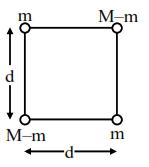# A body of mass`
Question:

A body of mass (2M) splits into four masses $\{\mathrm{m}, \mathrm{M}-\mathrm{m}, \mathrm{m}, \mathrm{M}-\mathrm{m}\}$, which are rearranged to form a square as shown in the figure. The ratio of $\frac{\mathrm{M}}{\mathrm{m}}$ for which, the gravitational potential energy of the system becomes maximum is $x: 1$. The value of $x$ is .......Solution:

Energy is maximum when mass is split equally so

$\frac{M}{m}=2$